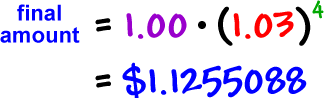Let's test it on the last one we figured out:

If we invest \$1.00 in an account that pays 12% compounded  quarterly, how much will we have in the account at the end of one year?

initial amount = \$1.00

At the end of each period (quarter), we'll be earning 3%...

So, each \$1.00 will turn into \$1.03

growth factor = \$1.03

number of periods = 4

Here's the formula:So, with our numbers:Hey, it works!  We got the same amount that we got doing it the long way...  And this was a LOT easier.